﻿ DE Based Job Scheduling in Grid Environments

### DE Based Job Scheduling in Grid Environments

Ch.Srinivasa Rao, Dr.B.Raveendra BabuOPEN ACCESSPEER-REVIEWED

## DE Based Job Scheduling in Grid Environments

Ch.Srinivasa Rao1,, Dr.B.Raveendra Babu2

1R.V.R. & J.C. College of Engineering, Guntur, A.P., India

2VNR Vignana Jyothi Institute of Engineering & Technology, Hyderabad, A.P., India

### Abstract

Grid Computing is a computing framework developed to meet the growing computational demands. Essential grid services contain more intelligent functions for resource management, grid service marketing, collaboration etc. The load sharing of computational jobs is the major task of computational grids. Grid resource manager provides functional mechanism for discovery, publishing of resources as well as scheduling, submission and monitoring of jobs. This paper introduces an approach, based on Differential Evolution Algorithm for scheduling jobs on computational grid. The proposed approach generates an optimal schedule which helps in completing the jobs within a minimum period of time. We evaluate the performance of our proposed approach with a direct Genetic Algorithm (GA), Simulated Annealing (SA) and Particle Swarm Optimization (PSO) approach.

### At a glance: Figures

1
Prev Next

• Rao, Ch.Srinivasa, and Dr.B.Raveendra Babu. "DE Based Job Scheduling in Grid Environments." Journal of Computer Networks 1.2 (2013): 28-31.
• Rao, C. , & Babu, D. (2013). DE Based Job Scheduling in Grid Environments. Journal of Computer Networks, 1(2), 28-31.
• Rao, Ch.Srinivasa, and Dr.B.Raveendra Babu. "DE Based Job Scheduling in Grid Environments." Journal of Computer Networks 1, no. 2 (2013): 28-31.

 Import into BibTeX Import into EndNote Import into RefMan Import into RefWorks

### 1. Introduction

Grid Computing is a form of distributed computing that involves coordinating and sharing computing, application, data and storage or network resources across dynamic and geographically dispersed organization . Grid technologies promise to change, the way organizations tackle complex computational problems. Grid computing is an evolving area of computing where standards and technology are still being developed to enable this new paradigm.

Users can share grid resources by submitting computing tasks to grid system. The resources of computational grid are dynamic and belong to different administrative domains. The participation of resources can be active or inactive within the grid. Hence, it is impossible for anyone to manually assign jobs to computing resources in grids. Therefore grid job scheduling is one of the challenging issues in grid computing. Grid scheduling system selects the resources and allocates the user submitted jobs to appropriate resources in such a way that the user and application requirements are met.

There are many research efforts aiming at job scheduling on the grid. Scheduling m jobs to n resources with an objective to minimize the total execution time has been shown to be NP-complete . Therefore the use of heuristics is the defacto approach in order to cope in practice with its difficulty. Krauter et al. provided a useful survey on grid resource management systems, in which most of the grid schedulers such as AppLes, Condor, Globus, Legion, Netsolve, Ninf and Nimrod use simple batch scheduling heuristics . Jarvis et al. proposed the scheduling algorithm using metaheuristics and compared FCFS with genetic algorithm to minimize the makespan and it was found that metaheuristics generate good quality schedules than batch scheduling heuristics . Braun et al. studied the comparison of the performance of batch queuing heuristics, tabu search, genetic algorithm and simulated annealing to minimize the makespan . The results revealed that genetic algorithm achieved the best results compared to batch queuing heuristics. Hongbo Liu et al. proposed a fuzzy particle swarm optimization (PSO) algorithm for scheduling jobs on computational grid with the minimization of makespan as the main criterion . They empirically showed that their method outperforms the genetic algorithm and simulated annealing approach. The results revealed that the PSO algorithm has an advantage of high speed of convergence and the ability to obtain faster and feasible schedules.

In this paper, we address a job scheduling problem on computational grid, in which minimization of execution time is considered as the objective. To tackle this problem, Differential Evolution algorithm is proposed to search for the optimal solution, to complete the batch of jobs in a minimum period of time.

The rest of the paper is organized as follows: Section 2 presents the problem statement related to job scheduling. In Section 3, background of DE algorithm is described and the proposed algorithm is outlined. The computational results are reported in Section 4 and the conclusions are presented in Section 5.

### 2. Problem Statement

Scheduling is the process of mapping the jobs to specific time intervals of the grid resources. The grid job scheduling problem consists of scheduling m jobs with given processing time on n resources. Let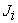be the independent user jobs,. Let Ri be the heterogeneous resources,. The speed of each resource is expressed in number of cycles per unit time (CPUT). The length of each job is expressed in number of cycles. The information related to job length and speed of the resource is assumed to be known, based on user supplied information, experimental data and application profiling or other techniques .

The objective of the proposed job scheduling algorithm is to minimize the makespan. Makespan is a measure of the throughput of the heterogeneous computing system. Let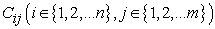be the completion time that the resource Ri finishes the job,represents the time that the resource Ri finishes all the jobs scheduled for itself. Makespan is defined as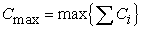.

Any job Jj has to be processed in resource Ri until completion.

Jobs come in batch mode.

All jobs and grid resources are submitted at once before the start of processing each batch.

### 3. Scheduling Using DE

Differential Evolution is a novel population based evolutionary algorithm, which has been proposed for optimizing complex problems over a continuous domain. DE searches for the global optima by utilizing differences between contemporary population members, which allows the search behavior of each individual to self-tune. So far, DE has attracted much attention and wide applications in a variety of fields [8, 9].

Onwubolu et al. addressed the flow-shop scheduling problem using DE algorithm . In their work, the algorithm was implemented by mapping Job/Machine sequence to real numbers for DE operations. Since this approach is not feasible in the case of grid scheduling, Talukder et al. proposed a workflow execution planning approach using Multi objective Differential Evolution to generate trade-off schedule by considering the completion time of tasks and the total execution cost of jobs, in which they dealt with exact scheduling sequences . Our approach makes use of integer values in order to map the resource/job sequence.

3.1. Differential Evolution Algorithm

The differential evolution algorithm (DE) introduced by Storn and Price is a novel parallel direct search method, which utilizes NP parameter vectors as a population for each generation G. DE is a kind of evolutionary optimization algorithm. There are several variants of DE available . This paper makes use of the DE/rand/1/bin scheme.

It starts with the random initialization of the initial population of NP individuals. Each individual has an n dimensional vector. The ith individual at generation ‘t’ can be represented as.

According to the mutation operator, a mutant vector is generated by adding the weighted difference between two randomly selected target population individuals to a third individual as follows.(1)

Where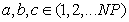are randomly chosen and mutually exclusive.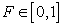is the scaling factor which affects the differential variation between two individuals. After the mutation phase, the cross over operator is applied to obtain the trail vector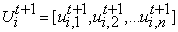by the following equation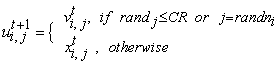(2)

Where randj is the jth independent random number uniformly distributed in the range of [0,1][, 1]. Also randni refers to a randomly chosen index from the set {1, 2…n}.CR is a user defined cross over factor in the range [0,1][, 1].

Following the crossover operation, to decide whether or not the trail vectorshould be a member of the population of the next generation, it is compared with the target individual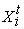Finally the selection is based on the survival of the fitness as follows.(3)
3.2. The Proposed Job Scheduling Algorithm

In this section, we proposed a DE based Grid Job Scheduling Algorithm and presented a solution representation.

3.2.1. General Scheme of de Based Grid Job Scheduling Algorithm

The pseudo code for DE based grid job scheduling algorithm is illustrated in Algorithm 1. Table 1 depicts the explanation of abbreviated parameters used in Algorithm 1.

Algorithm 1 Grid Job Scheduling

Algorithm using DE

Define RT, JT, ESR, JL, F, CR, NP, MaxIter,

STR, ETR

Create the initial population of random individuals. Check the feasibility of initial population vectors

for 1 to MaxIter

Calculate the makespan of each individual

for i = 1 to NP

Select random integer randni ∈ (0, 1, 2... JT)

Select mutually exclusive random individuals Xa, Xb and Xc

Calculate mutant vector V according to equation (1) starting from the position randni of each individual.

Select the random value randj ∈[0, 1][, 1]

Calculate the trail vector Ui according to equation (2)

Check the feasibility of trail vector Ui

end for

Calculate the makespan of trail vector set

for i = 1 to NP

if makespan of Ui is less than Xi then

Select Ui

else

Retain Xi

end if

end for

Record the solution with minimum makespan

end for

#### Table 1.Parameters used in Algorithm 1

3.2.2. Solution Representation

In the proposed scheduling algorithm, the solution is represented as an array of length equal to the number of jobs. The value corresponding to each position i in the array represent the resource to which job i was allocated. The job-to-resource representation for the resource job pair (3, 13) is illustrated in Figure 1.

The first element of the array denotes the first job (J1) in a batch which is allocated to the Grid resource 2; the second element of the array denotes the second job (J2) which is assigned to the Grid resource 1, and so on ( see Figure 2)

### 4. Experiment Settings, Results and Discussions

In our experiments, Genetic Algorithm (GA) [4, 5], Simulated Annealing (SA)  and Particle Swarm Optimization(PSO)  were used to compare the performance with Differential Evolution(DE). The four algorithms share many similarities. The performance of the proposed scheduling algorithm was tested for the resource job pairs of small scale problem (3,13) and large scale problems such as (5,100), (8,60) and (10,50). The numerical simulations are carried out with the dataset used and tested in the paper.

The DE based grid job scheduling algorithm is coded in MATLAB R2008a and experiments are executed on a Pentium IV 2.99 GHz PC with 1 GB memory.

To illustrate the algorithm, we considered a finite number of grid nodes and assumed that the processing speeds of the grid nodes (cput) and the job lengths (processing requirements in cycles) are known. Each experiment (for each algorithm) was repeated 10 times with different random seeds. Each trial had a fixed number of 50*m*n iterations (m is the number of the grid nodes, n is the number of the jobs). The makespan values of the best solutions throughout the optimization run were recorded.

In order to closely track the performance of our algorithms, first we tested a small scale job scheduling problem, (3,13), in which 3 nodes and 13 jobs are listed in Figure 1. The results for 10 GA runs were {47, 46, 47, 47.3333, 46, 47, 47, 47, 47.3333, 49}, with an average value of 47.1167. The results of 10 SA runs were {46.5, 46.5, 46, 46, 46, 46.6667, 47, 47.3333, 47, 47} with an average value of 46.6. The results of 10 PSO runs were {46, 46, 46, 46, 46.5, 46.5, 46.5, 46, 46.5, 46.6667}, with an average value of 46.2667. The results of 10 DE runs were {46, 46, 46, 46.5, 46, 46, 46, 46, 46, 46}, with an average value of 46.05. The optimal result is supposed to be 46.05. Further, we tested the four algorithms for other three (G; J) pairs, i.e. (5,100), (8, 60) and (10, 50). All the jobs and the nodes were submitted at one time. The average makespan values for 10 trials are showed in Table 2. DE had better average makespan values than the other three algorithms for resource job pairs (3,13) and (10,50). The experimental results are illustrated in Figure 3.

### 5. Conclusions

This paper presents a DE based job scheduling approach to solve grid scheduling problem to minimize the completion time. The proposed scheduling algorithm is very simple, as it involves small number of parameters for devising the algorithm. As the status of resource is dynamic within the grid environment, it is necessary to produce the faster and feasible schedules. In our future work, it is proposed to develop adaptive DE based algorithms and generalize the DE-based algorithm to multi-objective complex scheduling problems and stochastic scheduling problems.

### Acknowledgements

We would like to thank, Professor A.Abraham for providing the datasets to implement and test the algorithm proposed in this paper. The work is supported by Professor K.Karteeka Pavan.

### References

  I.Foster,C.Kesselmann,(Eds.), The Grid: Blueprint for a New Computing Infrastructure, Morgan Kaufmann Publishers, , 1999.In article  O.H.Ibarra and C.E.Kim, Heuristic algorithms for scheduling independent tasks on nonidentical processors, J.ACM 24, 2 (Apr.1977), pp.280-289.In article CrossRef  K.Krauter, R.Buyya, M.Maheswaran,A taxonomy and survey of grid resource management systems for distributed computing, Software-Practice and Experience,32:135-164, 2002.In article CrossRef  S.A.Jarvis, D.P.Spooner, H.N.Lim Choi Keung, G.R.Nudd, J.Cao, S.Saini, Performance prediction and its use in parallel and distributed computing systems. In the Proceedings of the IEEE/ACM International Workshop on Performance Evaluation and Optimization of Parallel and distributed Systems, Nice, France.2003.In article  T.D.Braun, H.J.Siegel, N.Beck, D.A.Hensgen, R.F.Freund, A comparison of eleven static heuristics for mapping a class of independent tasks on heterogeneous distributed systems, Journal of Parallel and Distributed Computing, 2001, pp.810-837.In article CrossRef  H.Liu, A.Abraham, A.E.Hassanien, Scheduling Jobs on computational grids using a fuzzy particle swarm optimization algorithm, Future Generation Computer Systems (2009).In article  S.K.Garg, R.Buyya, H.J.Siegel, Time and cost trade-off management for scheduling parallel applications on Utility Grids, Future Generation Computer Systems (2009).In article  F.P.Chang, C.Hwang, Design of digital PID Controllers for continuous time plants with performance criteria, Journal of the chineese Institute of Chemical Engineers, 35(6), 2004, pp.683-696.In article  Y.P.Chang, C.J.Wu, Optimal multiobjective planning of large-scale passive harmonic filters using hybrid differential evolution method considering parameter and loading uncertainty, IEEE transactions on Power Delivery, 20(1), 2005, pp.408-416.In article CrossRef  G.Onwubolu, D.Davendra, Discrete Optmization Scheduling flow shops using differential evolution algorithm, European Journal of Operational Research 171 2006, pp.674-692.In article CrossRef  A.K.M.K.A.Talukder, M.Kirley, R.Buyya, Multi objective Differential Evolution for Scheduling workflow applications on global Grids, Concurrency and Computation: Practice and Experience, published online in Wiley Interscience, (2009).In article  K.Price, R.Storn, Differential evolution (DE) for Continuous function optimization, 2007.http://www.icsi.berkeley.edu/%7Estorn/code.html.In article